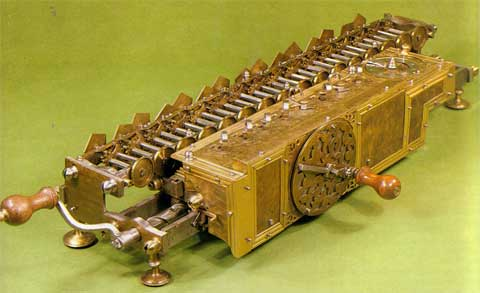### From SU(1,1) to the Lorentz group

From the two-dimensional disk we are moving to three-dimensional space-time. We will meet Einstein-Poincare-Minkowski special relativity, though in a baby version, with x and y, but without z in space. It is not too bad, because the famous Lorentz transformations, with length contraction and time dilation happen already in two-dimensional space-time, with x and t alone. We will discover Lorentz transformations today. First in disguise, but then we will unmask them.

First we recall, from The disk and the hyperbolic model, the relation between the coordinateson the Poincare disk, andon the unit hyperboloid(1)(2)We have the group SU(1,1) acting on the disk with fractional linear transformations. Withandin SU(1,1)

(3)the fractional linear action is

(4)By the way, we know from previous notes thatis in SU(1,1) if and only if

(5)Having the new point on the disk, with coordinateswe can use Eq. (1) to calculate the new space-time point coordinatesThis is what we will do now. We will see that even ifdepends onin a nonlinear way, the space-time coordinates transform linearly. We will calculate the transformation matrixand express it in terms ofandWe will also check that this is a matrix in the group SO(1,2).

The program above involves algebraic calculations. Doing them by hand is not a good idea. Let me recall a quote from Gottfried Leibniz, who, according to Wikipedia

He became one of the most prolific inventors in the field of mechanical calculators. While working on adding automatic multiplication and division to Pascal’s calculator, he was the first to describe a pinwheel calculator in 1685 and invented the Leibniz wheel, used in the arithmometer, the first mass-produced mechanical calculator. He also refined the binary number system, which is the foundation of virtually all digital computers.“It is unworthy of excellent men to lose hours like slaves in the labor of calculation which could be relegated to anyone else if machines were used.”— Gottfried Leibniz

I used Mathematica as my machine. The same calculations can be certainly done with Maple, or with free software like Reduce or Maxima. For those interested, the code that I used, and the results can be reviewed as a separate HTML document: From SU(1,1) to Lorentz.

Here I will provide only the results. It is important to notice that while the matrixhas complex entries, the matrixis real. The entries ofdepend on real and imaginary parts ofand(6)Here is the calculated result for:

(7)In From SU(1,1) to Lorentz it is first verified that the matrixis of determinant 1. Then it is verified that it preserves the Minkowski space-time metric. Withdefined as

(8)we have

(9)Sincethe transformationpreserves the time direction. Thusis an element of the proper Lorentz group.

Remark: Of course we could have chosenwithon the diagonal. We would have the group SO(2,1), and we would write the hyperboloid asIt is a question of convention.

In SU(1,1) straight lines on the disk we considered three one-parameter subgroups of SU(1,1):

(10)(11)We can now use Eq. (7) in order to see which space-time transformations they implement. Again I calculated it obeying Leibniz and using a machine (see From SU(1,1) to Lorentz).A replica of the Stepped Reckoner of Leibniz form 1923 (original is in the Hannover Landesbibliothek)

Here are the results of the machine work:

(12)(13)(14)The third family is a simple Euclidean rotation in theplane. That is why I denoted the parameter with the letterIn order to “decode” the first two one-parameter subgroups it is convenient to introduce new variableand setThe group propertyis then lost, but the matrices become evidently those of special Lorentz transformations,transformingand, leavingunchanged, andtransformingand leavingunchanged (though with a different sign of). Taking into account the identities

(15)we get

(16)(17)In the following posts we will use the relativistic Minkowski space distance on the hyperboloid for finding the distance formula on the Poincare disk.